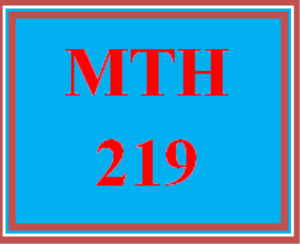# MTH 219 Week 1 Introductory & Intermediate Algebra for College Students, Ch. 1, Sections 1.1, 1.4, & 1.8: Introduction to Algebra: Variables and Mathematical Models

PLDZ-6412 In Stock
\$ 2.00 USD
Description

MTH 219 Week 1 Introductory & Intermediate Algebra for College Students, Ch. 1, Sections 1.1, 1.4, & 1.8: Introduction to Algebra: Variables and Mathematical Models

https://zh.scribd.com/document/343514419/Uop-Tutorial

# MTH 219 Week 1 Introductory & Intermediate Algebra for College Students, Ch. 1, Sections 1.1, 1.4, & 1.8: Introduction to Algebra: Variables and Mathematical Models

Learning Mathematics is like learning a new language. In this chapter you learn how to use the English language to write equations and expressions. Once you have gotten the hang of using English words to translate into mathematics you will check to see if a given number is the answer to a problem.

Your Ch. 1 reading also supports your Week 1 MyMathLab® assignment. The reading provides you step by step examples in case you get stuck or lost in MyMathLab®.

Recent Reviews Write a Review
0 0 0 0 reviews